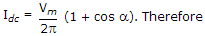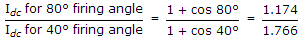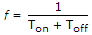# Electronics and Communication Engineering - Power Electronics

### Exercise :: Power Electronics - Section 1

11.

An SCR is triggered at 40° in the positive half cycle only. The average anode current is 50 A. If the firing angle is changed to 80°, the average anode current is likely to be

 A. 50 A B. 25 A C. less than 50 A but more than 25 A D. less than 25 A

Explanation:.

12.

The characteristics of a non-linear resistance is i = kv4. If i becomes 100 times, v becomes

Explanation:

v ∝ (i)1/4 ∝ (100)1/4 ≈ 3.

13.

In a step down chopper using pulse width modulation, Ton = 3 x 10-3 and Toff = 1 x 10-3 s. The chopping frequency is

 A. 333.33 B. 250 C. 500 D. 1000

Explanation:.

14.

Thyristors are suitable for dc circuit breakers but not for ac circuit breakers.

 A. True B. False

Explanation:

They are suitable for both dc and ac circuit breakers.

15.

Assertion (A): Inverters and choppers use fast switching thyristors

Reason (R): Fast switching SCR has low turn off time.

 A. Both A and R are correct and R is correct explanation of A B. Both A and R correct but R is not correct explanation of A C. A is correct but R is wrong D. A is wrong but R is correct